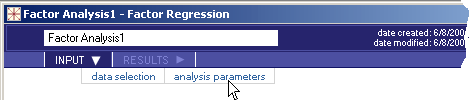# 5.4.5 - Factor Analysis - INPUT - Analysis Parameters - Teradata Warehouse Miner

## Teradata Warehouse Miner User Guide - Volume 3Analytic Functions

Product
Release Number
5.4.5
Published
February 2018
Language
English (United States)
Last Update
2018-05-04
dita:mapPath
yuy1504291362546.ditamap
dita:ditavalPath
ft:empty
1. On the Factor Analysis dialog box, click INPUT.
2. Click analysis parameters.
Factor Analysis > Input > Analysis Parameters3. On this screen, select:
• General Options
• Analysis method
• Principal Components (PCA) — As described above. This is the default method.
• Principal Axis Factors (PAF) — As described above.
• Maximum Likelihood Factors (MLF) — As described above.
• Convergence Method
• Minimum Eigenvalue

PCA — Minimum eigenvalue to include in principal components (default 1.0)

MLF — Option does not apply (N/A)

• Number of Factors — The user may request a specific number of factors as an alternative to using the minimum eigenvalue option for PCA and PAF. Number of factors is however required for MLF. The number of factors requested must not exceed the number of requested variables.
• Convergence Criterion
• PCA — Convergence criterion does not apply
• PAF — Iteration continues until maximum communality change does not exceed convergence criterion
• MLF — Iteration continues until maximum change in the square root of uniqueness values does not exceed convergence criterion
• Maximum Iterations
• PCA — Maximum iterations does not apply (N/A)
• PAF — The algorithm stops if the maximum iterations is exceeded (default 100)
• MLF — The algorithm stops if the maximum iterations is exceeded (default 1000)
• Matrix Type — The product automatically converts the extended cross-products matrix stored in metadata results tables by the Build Matrix function into the desired covariance or correlation matrix. The choice will affect the scaling of resulting factor measures and factor scores.
• Correlation — Build a correlation matrix as input to Factor Analysis. This is the default option.
• Covariance — Build a covariance matrix as input to Factor analysis.
• Invert signs if majority of matrix values are negative (check box) — You may optionally request that the signs of factor loadings and related values be changed if there are more minus signs than positive ones. This is purely cosmetic and does not affect the solution in a substantive way. Default is enabled.
• Rotation Options
• Rotation Method
• None — No factor rotation is performed. This is the default option.
• Varimax — Gamma in rotation equation fixed at 1.0. The varimax criterion seeks to simplify the structure of columns or factors in the factor loading matrix.
• Quartimax — Gamma in rotation equation fixed at 0.0. the quartimax criterion seeks to simplify the structure of the rows or variables in the factor loading matrix.
• Equamax — Gamma in rotation equation fixed at f / 2.
• Parsimax — Gamma in rotation equation fixed at v(f-1) / (v+f+2).
• Orthomax — Gamma in rotation equation set by user.
• Quartimin — Gamma in rotation equation fixed at 0.0. Provides the most oblique rotation.
• Biquartimin — Gamma in rotation equation fixed at 0.5.
• Covarimin — Gamma in rotation equation fixed at 1.0. Provides the least oblique rotation.
• Orthomin — Gamma in rotation equation set by user.
• Report Options
• Variable Statistics — This report gives the mean value and standard deviation of each variable in the model based on the derived SSCP matrix.
• Near Dependency — This report lists collinear variables or near dependencies in the data based on the derived SSCP matrix.
• Condition Index Threshold — Entries in the Near Dependency report are triggered by two conditions occurring simultaneously. The one that involves this parameter is the occurrence of a large condition index value associated with a specially constructed principal factor. If a factor has a condition index greater than this parameter’s value, it is a candidate for the Near Dependency report. A default value of 30 is used as a rule of thumb.
• Variance Proportion Threshold — Entries in the Near Dependency report are triggered by two conditions occurring simultaneously. The one that involves this parameter is when two or more variables have a variance proportion greater than this threshold value for a factor with a high condition index. Another way of saying this is that a ‘suspect’ factor accounts for a high proportion of the variance of two or more variables. This parameter defines what a high proportion of variance is. A default value of 0.5 is used as a rule of thumb.
• Collinearity Diagnostics Report — This report provides the details behind the Near Dependency report, consisting of the “Eigenvalues of Unit Scaled X’X”, “Condition Indices” and “Variance Proportions” tables.
• Long Report — This option, when checked, causes numerous detail and intermediate reports to be produced, as described in Factor Analysis - RESULTS - Reports.